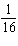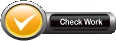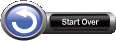Name:    3rd Grade GeometryReason with shapes and their attributes.  Standard 2, Test 1

Multiple Choice
Identify the choice that best completes the statement or answers the question.

1.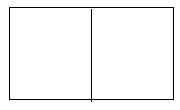Which fraction below describes how this rectangle is divided?
 a.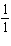c.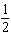b.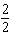d. 1

2.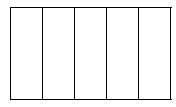Which fraction below describes how this rectangle is divided?
 a.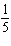c. 1 b.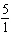d. 5

3.Which fraction below describes how this rectangle is divided?
 a.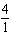c.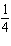b.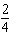d. 4

4.Which fraction below describes how this shape is divided?
 a.c.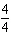b.d.5.Which fraction below describes how this shape is divided?
 a.c.b.d.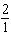6.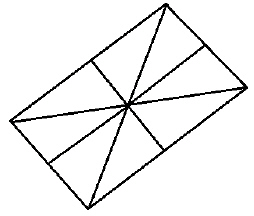Which fraction below describes how much each part of this shape is divided?
 a.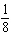c.b.d.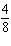7.Which fraction below describes into how much each part of this shape is divided?
 a.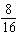c.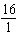b.d.